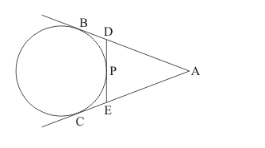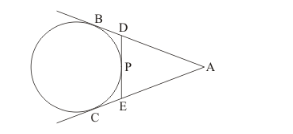# In the given figure, if AB = 8 cm and PE = 3 cm, then AE =

Question:

In the given figure, if AB = 8 cm and PE = 3 cm, then AE =(a) 11 cm

(b) 7 cm

(c) 5 cm

(d) 3 cm

Solution:

We know that tangents drawn from the same external point will be equal in length.Therefore,

AB = AC

It is given that,

AB = 8 cm

Hence,

AC = 8 cm …… (1)

Similarly,

PE = CE

It is given that,

PE = 3

Therefore,

CE = 3 …… (2)

Subtracting equations (1) and (2), we get,

AC − CE = 8 − 3

From the figure we can see that,

AC − CE = AE

Therefore,

AE = 8 − 3

AE = 5 cm

Option (c) is the correct answer.